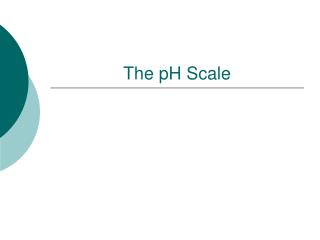DownloadDownload PresentationThe pH Scale

# The pH Scale

Download Presentation## The pH Scale

- - - - - - - - - - - - - - - - - - - - - - - - - - - E N D - - - - - - - - - - - - - - - - - - - - - - - - - - -
##### Presentation Transcript

1. The pH Scale

2. What does pH stand for? potential Hydrogen Refers to the substance’s ability to attract Hydrogen atoms

3. The pH Scale • A series of numbers from 1 to 14. • Used to describe how acidic or basic a solution is. • Most acidic substances are at the low end of the scale. • Most basic substances are at the high end of the scale.

4. pH Indicator Paper • You can find the pH of a solution by using indicator paper or an indicator solution. • Indicator paper/solution turns a different color for each pH value. • Matching the color of the paper/solution with the colors on the scale tells how acidic or basic a solution is.

5. Neutral pH • A solution with a pH of 7 is neutral. This means that the solution is neither acidic nor basic. • Example: Pure water has a pH of 7.

6. Basic pH • Basic solutions have a pH greater than 7. • High ability to attract Hydrogen ions. • Example: Soap has a pH of 10.

7. Acidic pH • Solutions with a pH less than 7 are acidic. • Low ability to attract Hydrogen ions. • Example: Lemons have a pH of 2.

8. Sample pH Scale • Number the line (left to right) from 1 to 14 (spaced evenly).